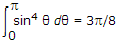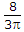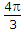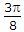# Electronics and Communication Engineering - Electromagnetic Field Theory

6.
Given that, if the radiation intensity of an antenna is proportional to sin2 θ, where θ is the elevation angle, the antenna directivity will beExplanation:
No answer description is available. Let's discuss.

7.
The term critical frequency denotes
the lowest frequency that is reflected by the ionosphere
the highest frequency that is reflected by the ionosphere at vertical distance
the lowest frequency by which communication is possible between two given points
none of the above
Explanation:
No answer description is available. Let's discuss.

8.
The important postulate arising out of Maxwell's modification of Ampere's law is
a changing magnetic field produces an electric field
a changing electric field produces a magnetic field
a motional e.m.f. is produced by a coil moving in a magnetic field
a steady current produce a magnetic field
Explanation:
No answer description is available. Let's discuss.

9.
Which of the following is not a wideband antenna?
Marconi
Discone
Helical
Folded dipole